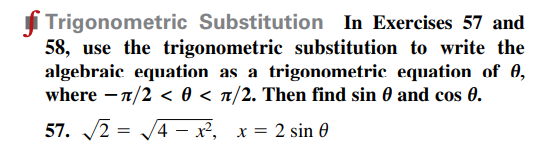### Still have math questions?

Trigonometry
Questionfrigonometric Substitution In Exercises $$57$$ and

$$58$$ , use the trigonometric substitution to write the algebraic equation as a trigonometric equation of $$\theta$$ , where $$- \pi / 2 < \theta < \pi / 2$$ . Then find $$\sin \theta$$ and $$\cos \theta$$ . 57. $$\sqrt { 2 } = \sqrt { 4 - x ^ { 2 } } , x = 2 \sin \theta$$

57 ) $$cos\theta= \frac{1}{\sqrt{8} }$$